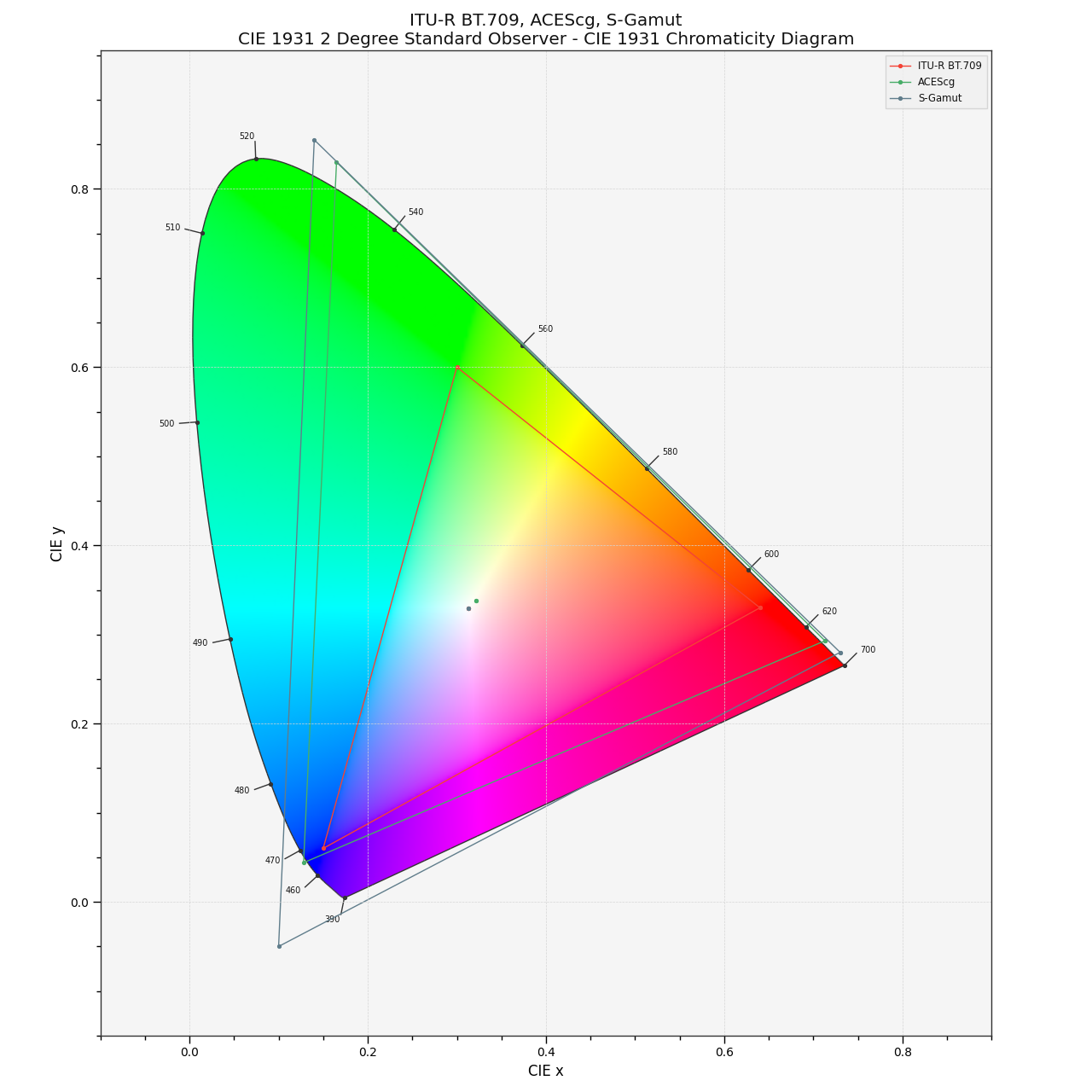colour.plotting.plot_RGB_colourspaces_in_chromaticity_diagram_CIE1931¶

colour.plotting.plot_RGB_colourspaces_in_chromaticity_diagram_CIE1931(colourspaces=None, cmfs='CIE 1931 2 Degree Standard Observer', chromaticity_diagram_callable_CIE1931=<function plot_chromaticity_diagram_CIE1931>, **kwargs)[source]

Plots given RGB colourspaces in the CIE 1931 Chromaticity Diagram.

Parameters: Other Parameters: colourspaces (array_like, optional) – RGB colourspaces to plot. cmfs (unicode, optional) – Standard observer colour matching functions used for Chromaticity Diagram bounds. chromaticity_diagram_callable_CIE1931 (callable, optional) – Callable responsible for drawing the CIE 1931 Chromaticity Diagram. **kwargs (dict, optional) – {colour.plotting.artist(), colour.plotting.diagrams.plot_chromaticity_diagram(), colour.plotting.render()}, Please refer to the documentation of the previously listed definitions. Current figure and axes. tuple

Examples

>>> plot_RGB_colourspaces_in_chromaticity_diagram_CIE1931(
...     ['ITU-R BT.709', 'ACEScg', 'S-Gamut'])
... # doctest: +SKIP